true
true

Anjan Debnath

East Kolkata Township, Kolkata, India - 700107# Anjan Debnath

East Kolkata Township, Kolkata, India - 700107.

Referral Discount: Get ₹ 500 off when you make a payment to start classes. Get started by Booking a Demo.

Details verified of Anjan Debnath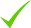IdentityEducation

Know how UrbanPro verifies Tutor details

Identity is verified based on matching the details uploaded by the Tutor with government databases.

## Overview

First of all, I am an Assistant Professor and HOD, Department of Mathematics, The Heritage College, Kolkata. Also, I am a mathematician working my PhD in Number Theory,a branch of higher mathematics,acter completing my MPhil from Ramanujan Institute.

When I teach mathematics my students, they certify the following, "we never knew one can teach this subject so lucidly and beautifully that one will feel or start getting to feel.confident to tackle the problems".

In my 9 years of teaching expereince, i have understood the following. a student feels mathematics is tough or difficult because the way in regular syllabus they are taught is mechanical. in my class, i show the students to look around and explore the ideas in mathematics. i always encourage them to push beyond their limit so that they can loose the fear of mathematics. Because of which, students who got failed twice in university exam, cleared it in third attempt after my training.

sincerity, dedication and passionate about teaching and learning the subject, to interact with the students to understand their queries, their difficulties in mathematics are my nature. check.up home work on regular basis, conducing monthly test in order to maintain the progress and improvement etc are also done by me regularly.

i always welcome the parents and the students to clear their doubt. if it is necessary, i contact personally and make it clear to them.

## Languages Spoken

Bengali Mother Tongue (Native)

English Proficient

Hindi Proficient

## Education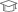Ramanujan Institue for Advanced Study in Mathematics Pursuing

Doctor of Philosophy (Ph.D.)Ramanujan institute for advanced study in mathematics 2013

Master of Philosophy (M.Phil.)

East Kolkata Township, Kolkata, India - 700107

## Verified Info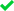ID VerifiedPhone VerifiedEmail Verified

Report this Profile

Is this listing inaccurate or duplicate? Any other problem?

Type the letters as shown below *

Please enter the letters as show below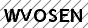Class 12 Tuition

Class LocationOnline (video chat via skype, google hangout etc)Student's HomeTutor's Home

Years of Experience in Class 12 Tuition

9

Board

IGCSE, ISC/ICSE, CBSE, State, International Baccalaureate

IB Subjects taught

Mathematics

ISC/ICSE Subjects taught

Mathematics

CBSE Subjects taught

Mathematics

IGCSE Subjects taught

Mathematics

Taught in School or College

Yes

State Syllabus Subjects taught

Mathematics

## Reviews

this is test message this is test message this is test message this is test message this is test message this is test message this is test message

No Reviews yet! Be the first one to Review

## FAQs

1. Which school boards of Class 12 do you teach for?

IGCSE, ISC/ICSE, CBSE and others

2. Have you ever taught in any School or College?

Yes

3. Which classes do you teach?

I teach BSc Tuition, Class 10 Tuition, Class 12 Tuition and Engineering Entrance Coaching Classes.

4. Do you provide a demo class?

Yes, I provide a free demo class.

5. How many years of experience do you have?

I have been teaching for 9 years.

## Answers by Anjan Debnath (2)

Dear Roshan.I have seen your query and as a maths professor, am trying my best to answer your doubt step by step, from my point of view and experience as well. First of all, as an instructor in mathematics,o i must advise you not to pay attention to neon thinking about this kind of questions. Your first... ...more

Dear Roshan.
I have seen your query and as a maths professor, am trying my best to answer your doubt step by step, from my point of view and experience as well.

First of all, as an instructor in mathematics,o i must advise you not to pay attention to neon thinking about this kind of questions. Your first and most essential nature should be to prepare yourself for the board exam with a bright concert in the subject matter. If you think about "how many questions might appear in exam outside the books" believe me or not, at the end of the day, somehow this will affect your mind, will make you frightened about the exam and eventually will change in your result. That's not the end. You might lose confidence in our preparation already you made. It means the portion you have studied and know that there will be a question from the part you studied, will become confusing after a while.

It is 100% guaranteed that a few questions will arrive in the exam paper which you will never find in the book. But what is important to notice or observe is that these questions will be entirely conceptual. On other words, you will be able to solve them only if your concept in subject matter is clear. Let me give you an example.

Say A is a matrix of order 3x3 given by
( [ 1,2,3 ], [0,2,3] , [ 0,0,3] )
Where I have written each row elements within square brackets. Means, 1,2,3 are the elements in the first row, 0,2,3 are the elements in second row etc. Suppose you are asked to find the determinant of A^4.

What will you do? Are you tempted to say, sir, I will find A^4 by using Axa and then A^2 x A^2 and then find the determinant ?! Well, that will do the job but imagine if they ask you the same question but for A^100. Then? You are not going to take the above approach, are you? So how to solve this one?

Simple. Observe that A is an upper triangular matrix and the determinant of the upper triangular matrix the product of the diagonal elements. In that way, determinant of A is 1x2x3, i.e. 6.

Now det(A^4)={ det(A) }^4. Hence the required answer will be 6^4. As you can see, the determinant of A^100 has now become easier as well.

The above question naturally clear that, you can tackle such question through a clear concept only. These kinds of questions will appear as question outside NCERT math book, and if your preparation and concepts are strong enough, you don't have to worry about the exam paper any more.

This is why I am advising you. Study the entire book. First, finish every exercise. Once you are done, move into the examples which are provided in every chapter just before the use. Study them. Sometimes question appears from this zone also, which most of the time, students do not bother to study and let me share a tip, several conceptual issues you can find are hidden here.

I hope I have addressed your query. But if you still have any doubt, let me know.

I wish you a bright future ahead.

Take care!

Dislike Bookmark

Yes, there are. I am trying to explain step by step.Say we want the square of n = 67. First of all, the square of 7 is 49. Write 9 in the answer place and keep 4 for carrying over process.∴n^2 = 9Then, see that 6 and 7 form the number 67. Their product is 42. The rule says you have to double it.... ...more

Yes, there are. I am trying to explain step by step.
Say we want the square of n = 67. First of all, the square of 7 is 49. Write 9 in the answer place and keep 4 for carrying over process.
∴n^2 = 9
Then, see that 6 and 7 form the number 67. Their product is 42. The rule says you have to double it. Hence you get double of 42 as 84. Also, you have 4 in your hand for carrying over. Together you get 84+4=88. Write the unit digit eight beside 9 and keep 10s digit 8 for next round carry-over process.
∴ n^2 = 89
Finally, the 10s digit is 6. Square it, and you will get 36. Plus you have 8 in your hand. Together we get 36+8, i.e. 44. Now write it beside 89, and we are done.
∴ 67^2 = 4489.
Try your self: 39^2, 23^2, 52^2.

For squaring three digits or more, there is procedure indeed, but I can't explain them here within short place.

Although I can show you some other special technique for squaring 2 digit numbers of special types.

1. If the number ends with 5:
Let's say we need to square 85. First, write 25 straight forward in answer place.
85^2 = 25

Then, note that after ignoring five we are left with the number 8 in the given number 85. The next number of 8 is 9. The rule says to make the product which is in our present case is 8 x 9, i.e. 72.

Write it down, and we are done.
85^2 = 7225.
Similarly, 95^2 = 9025 because 9 x 10 = 90 and 25 already is written etc.
115^2 = 13225 because 11 x 12 = 132. Etc

2. If the number is near to 100.
Let's say we need to square 93.

The difference between 93 and 100 is 7. The rule says to write down the square of 7 first, which is here 49.
Next, decrease 93 by 7. Which gives 86. Hence the final answer becomes
93^2=8649.
Similarly, 95^2 is 9025 because the difference between 95 and 100 is 5. The square of 5 is 25, and if we decrease 95 by 5, we shall get 90
Together we thus obtain
95^2= 9025.
Notice we have achieved the same already before by using the general squaring technique explained above.

Caution. Suppose we want to square 98. This time doesn't be tempted to write 964. That's not correct. While you are squaring the difference between 100 and the given number, make sure the answer occupies exactly two places only. If not, use 0 to fill up the lattice. Hence 98^2 is not 964 but in 9604.

Similarly, for 88^2, first, write down the square of the difference which is 12^2, i.e. 144. But this time it is a three-digit answer. So keep the last two digits and carry over the excess digit which is 1 in this present case. Now decrease 88 by 12, and we get 76. Added by carried over one we get 77. Hence 88^2 = 7744.

There are more so many special rules. But we can discuss them some other days.

Take care.

Dislike Bookmark
Class 12 Tuition 5.0

Class LocationOnline (video chat via skype, google hangout etc)Student's HomeTutor's Home

Years of Experience in Class 12 Tuition

9

Board

IGCSE, ISC/ICSE, CBSE, State, International Baccalaureate

IB Subjects taught

Mathematics

ISC/ICSE Subjects taught

Mathematics

CBSE Subjects taught

Mathematics

IGCSE Subjects taught

Mathematics

Taught in School or College

Yes

State Syllabus Subjects taught

Mathematics

Class 10 Tuition 5.0

Class LocationOnline (video chat via skype, google hangout etc)Student's HomeTutor's Home

Years of Experience in Class 10 Tuition

9

Board

CBSE, IGCSE, ICSE, State, International Baccalaureate

IB Subjects taught

Mathematics

CBSE Subjects taught

Mathematics

ICSE Subjects taught

Mathematics

IGCSE Subjects taught

Mathematics

Taught in School or College

Yes

State Syllabus Subjects taught

Mathematics

BSc Tuition 5.0

Class Location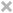Online (video chat via skype, google hangout etc)Student's HomeTutor's Home

Engineering Entrance Coaching classes 5.0

Class LocationOnline (video chat via skype, google hangout etc)Student's HomeTutor's Home

this is test message this is test message this is test message this is test message this is test message this is test message this is test message

No Reviews yet! Be the first one to Review

## Answers by Anjan Debnath (2)

Dear Roshan.I have seen your query and as a maths professor, am trying my best to answer your doubt step by step, from my point of view and experience as well. First of all, as an instructor in mathematics,o i must advise you not to pay attention to neon thinking about this kind of questions. Your first... ...more

Dear Roshan.
I have seen your query and as a maths professor, am trying my best to answer your doubt step by step, from my point of view and experience as well.

First of all, as an instructor in mathematics,o i must advise you not to pay attention to neon thinking about this kind of questions. Your first and most essential nature should be to prepare yourself for the board exam with a bright concert in the subject matter. If you think about "how many questions might appear in exam outside the books" believe me or not, at the end of the day, somehow this will affect your mind, will make you frightened about the exam and eventually will change in your result. That's not the end. You might lose confidence in our preparation already you made. It means the portion you have studied and know that there will be a question from the part you studied, will become confusing after a while.

It is 100% guaranteed that a few questions will arrive in the exam paper which you will never find in the book. But what is important to notice or observe is that these questions will be entirely conceptual. On other words, you will be able to solve them only if your concept in subject matter is clear. Let me give you an example.

Say A is a matrix of order 3x3 given by
( [ 1,2,3 ], [0,2,3] , [ 0,0,3] )
Where I have written each row elements within square brackets. Means, 1,2,3 are the elements in the first row, 0,2,3 are the elements in second row etc. Suppose you are asked to find the determinant of A^4.

What will you do? Are you tempted to say, sir, I will find A^4 by using Axa and then A^2 x A^2 and then find the determinant ?! Well, that will do the job but imagine if they ask you the same question but for A^100. Then? You are not going to take the above approach, are you? So how to solve this one?

Simple. Observe that A is an upper triangular matrix and the determinant of the upper triangular matrix the product of the diagonal elements. In that way, determinant of A is 1x2x3, i.e. 6.

Now det(A^4)={ det(A) }^4. Hence the required answer will be 6^4. As you can see, the determinant of A^100 has now become easier as well.

The above question naturally clear that, you can tackle such question through a clear concept only. These kinds of questions will appear as question outside NCERT math book, and if your preparation and concepts are strong enough, you don't have to worry about the exam paper any more.

This is why I am advising you. Study the entire book. First, finish every exercise. Once you are done, move into the examples which are provided in every chapter just before the use. Study them. Sometimes question appears from this zone also, which most of the time, students do not bother to study and let me share a tip, several conceptual issues you can find are hidden here.

I hope I have addressed your query. But if you still have any doubt, let me know.

I wish you a bright future ahead.

Take care!

Dislike Bookmark

Yes, there are. I am trying to explain step by step.Say we want the square of n = 67. First of all, the square of 7 is 49. Write 9 in the answer place and keep 4 for carrying over process.∴n^2 = 9Then, see that 6 and 7 form the number 67. Their product is 42. The rule says you have to double it.... ...more

Yes, there are. I am trying to explain step by step.
Say we want the square of n = 67. First of all, the square of 7 is 49. Write 9 in the answer place and keep 4 for carrying over process.
∴n^2 = 9
Then, see that 6 and 7 form the number 67. Their product is 42. The rule says you have to double it. Hence you get double of 42 as 84. Also, you have 4 in your hand for carrying over. Together you get 84+4=88. Write the unit digit eight beside 9 and keep 10s digit 8 for next round carry-over process.
∴ n^2 = 89
Finally, the 10s digit is 6. Square it, and you will get 36. Plus you have 8 in your hand. Together we get 36+8, i.e. 44. Now write it beside 89, and we are done.
∴ 67^2 = 4489.
Try your self: 39^2, 23^2, 52^2.

For squaring three digits or more, there is procedure indeed, but I can't explain them here within short place.

Although I can show you some other special technique for squaring 2 digit numbers of special types.

1. If the number ends with 5:
Let's say we need to square 85. First, write 25 straight forward in answer place.
85^2 = 25

Then, note that after ignoring five we are left with the number 8 in the given number 85. The next number of 8 is 9. The rule says to make the product which is in our present case is 8 x 9, i.e. 72.

Write it down, and we are done.
85^2 = 7225.
Similarly, 95^2 = 9025 because 9 x 10 = 90 and 25 already is written etc.
115^2 = 13225 because 11 x 12 = 132. Etc

2. If the number is near to 100.
Let's say we need to square 93.

The difference between 93 and 100 is 7. The rule says to write down the square of 7 first, which is here 49.
Next, decrease 93 by 7. Which gives 86. Hence the final answer becomes
93^2=8649.
Similarly, 95^2 is 9025 because the difference between 95 and 100 is 5. The square of 5 is 25, and if we decrease 95 by 5, we shall get 90
Together we thus obtain
95^2= 9025.
Notice we have achieved the same already before by using the general squaring technique explained above.

Caution. Suppose we want to square 98. This time doesn't be tempted to write 964. That's not correct. While you are squaring the difference between 100 and the given number, make sure the answer occupies exactly two places only. If not, use 0 to fill up the lattice. Hence 98^2 is not 964 but in 9604.

Similarly, for 88^2, first, write down the square of the difference which is 12^2, i.e. 144. But this time it is a three-digit answer. So keep the last two digits and carry over the excess digit which is 1 in this present case. Now decrease 88 by 12, and we get 76. Added by carried over one we get 77. Hence 88^2 = 7744.

There are more so many special rules. But we can discuss them some other days.

Take care.

Dislike Bookmark

Anjan Debnath conducts classes in BSc Tuition, Class 10 Tuition and Class 12 Tuition. Anjan is located in East Kolkata Township, Kolkata. Anjan takes at students Home, Regular Classes- at his Home and Online Classes- via online medium. He has 9 years of teaching experience . Anjan is pursuing Doctor of Philosophy (Ph.D.) from Ramanujan Institue for Advanced Study in Mathematics. Anjan has completed Master of Philosophy (M.Phil.) from Ramanujan institute for advanced study in mathematics in 2013. He is well versed in English, Hindi and Bengali.

•• Want to learn from Anjan Debnath?

• Contact Now
X

Recommended Profiles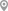Dhapa, KolkataRajendra Nagar, GhaziabadEast Kolkata Township, KolkataEast Kolkata Township, KolkataEast Kolkata Township, KolkataRaja Naba Krishna Street, Kolkata

X

Let us shortlist and give the best tutors and institutes.

or

Send Enquiry to Anjan Debnath

Let Anjan Debnath know you are interested in their class